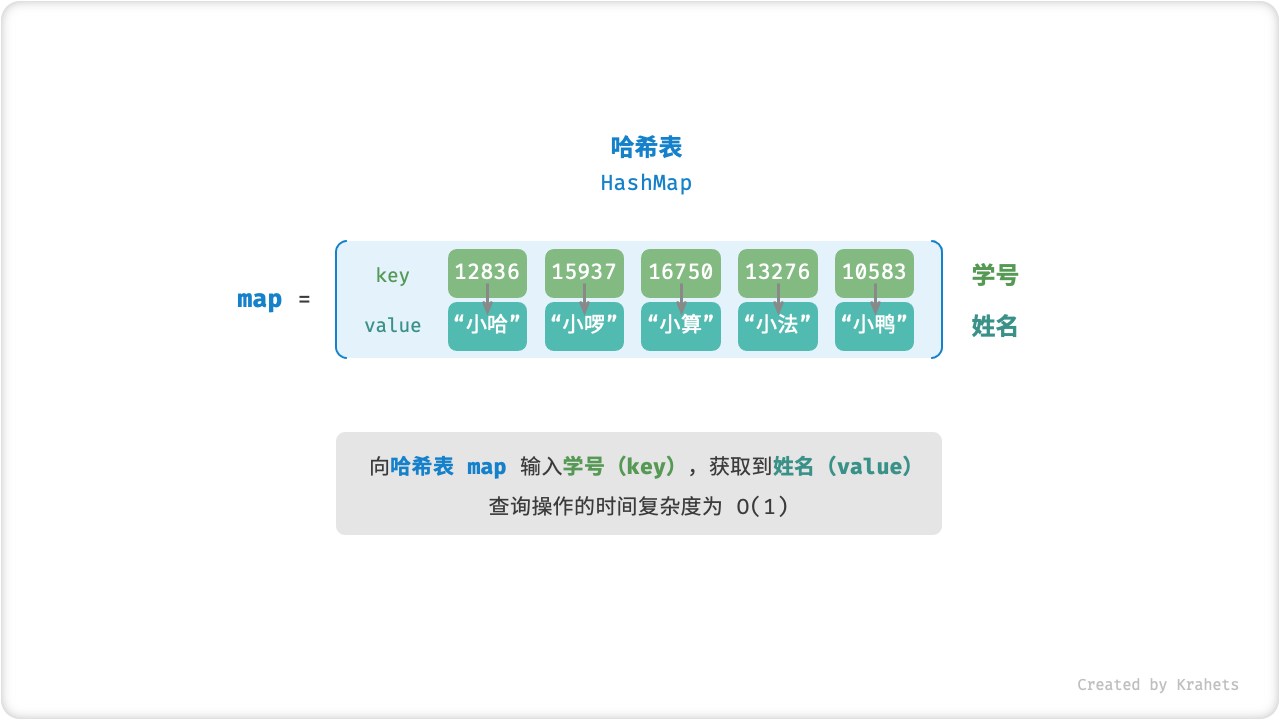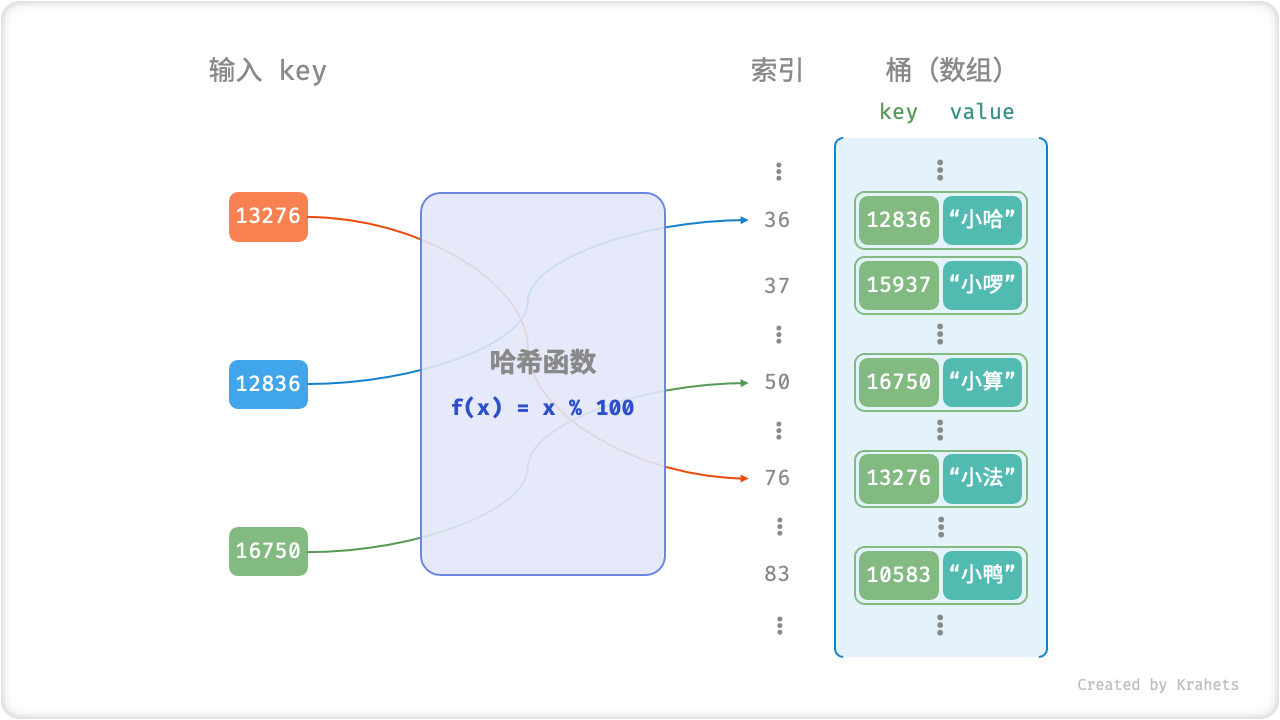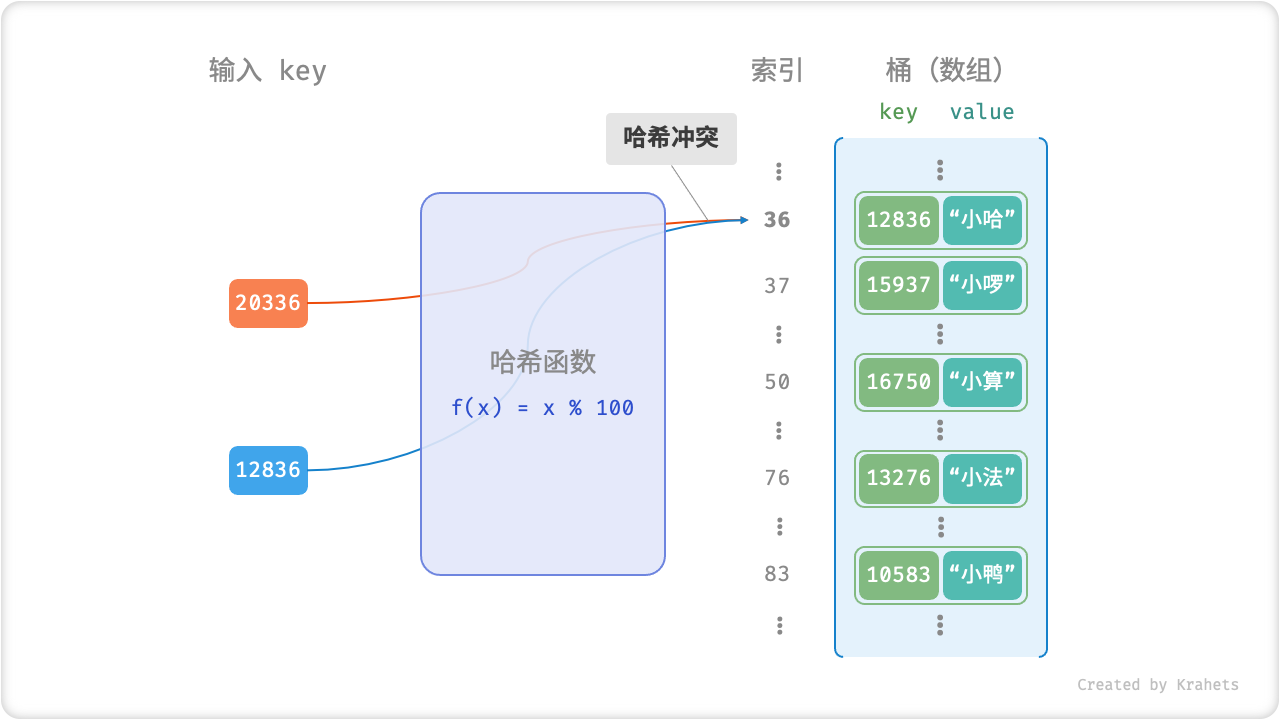# 哈希表¶Fig. 哈希表抽象表示

## 哈希表优势¶

1. 无序数组： 每个元素为 [学号, 姓名]
2. 有序数组：1. 中的数组按照学号从小到大排序；
3. 链表： 每个结点的值为 [学号, 姓名]
4. 二叉搜索树： 每个结点的值为 [学号, 姓名] ，根据学号大小来构建树；

## 哈希表常用操作¶

hash_map.java
/* 初始化哈希表 */
Map<Integer, String> map = new HashMap<>();

/* 添加操作 */
// 在哈希表中添加键值对 (key, value)
map.put(12836, "小哈");
map.put(15937, "小啰");
map.put(16750, "小算");
map.put(13276, "小法");
map.put(10583, "小鸭");

/* 查询操作 */
// 向哈希表输入键 key ，得到值 value
String name = map.get(15937);

/* 删除操作 */
// 在哈希表中删除键值对 (key, value)
map.remove(10583);

hash_map.cpp
/* 初始化哈希表 */
unordered_map<int, string> map;

/* 添加操作 */
// 在哈希表中添加键值对 (key, value)
map = "小哈";
map = "小啰";
map = "小算";
map = "小法";
map = "小鸭";

/* 查询操作 */
// 向哈希表输入键 key ，得到值 value
string name = map;

/* 删除操作 */
// 在哈希表中删除键值对 (key, value)
map.erase(10583);

hash_map.py
""" 初始化哈希表 """
mapp = {}

""" 添加操作 """
# 在哈希表中添加键值对 (key, value)
mapp = "小哈"
mapp = "小啰"
mapp = "小算"
mapp = "小法"
mapp = "小鸭"

""" 查询操作 """
# 向哈希表输入键 key ，得到值 value
name = mapp

""" 删除操作 """
# 在哈希表中删除键值对 (key, value)
mapp.pop(10583)

hash_map_test.go
/* 初始化哈希表 */
mapp := make(map[int]string)

/* 添加操作 */
// 在哈希表中添加键值对 (key, value)
mapp = "小哈"
mapp = "小啰"
mapp = "小算"
mapp = "小法"
mapp = "小鸭"

/* 查询操作 */
// 向哈希表输入键 key ，得到值 value
name := mapp

/* 删除操作 */
// 在哈希表中删除键值对 (key, value)
delete(mapp, 10583)

hash_map.js


hash_map.ts


hash_map.c


hash_map.cs



hash_map.java
/* 遍历哈希表 */
// 遍历键值对 key->value
for (Map.Entry <Integer, String> kv: map.entrySet()) {
System.out.println(kv.getKey() + " -> " + kv.getValue());
}
// 单独遍历键 key
for (int key: map.keySet()) {
System.out.println(key);
}
// 单独遍历值 value
for (String val: map.values()) {
System.out.println(val);
}

hash_map.cpp
/* 遍历哈希表 */
// 遍历键值对 key->value
for (auto kv: map) {
cout << kv.first << " -> " << kv.second << endl;
}
// 单独遍历键 key
for (auto key: map) {
cout << key.first << endl;
}
// 单独遍历值 value
for (auto val: map) {
cout << val.second << endl;
}

hash_map.py
""" 遍历哈希表 """
# 遍历键值对 key->value
for key, value in mapp.items():
print(key, "->", value)
# 单独遍历键 key
for key in mapp.keys():
print(key)
# 单独遍历值 value
for value in mapp.values():
print(value)

hash_map_test.go
/* 遍历哈希表 */
// 遍历键值对 key->value
for key, value := range mapp {
fmt.Println(key, "->", value)
}
// 单独遍历键 key
for key := range mapp {
fmt.Println(key)
}
// 单独遍历值 value
for _, value := range mapp {
fmt.Println(value)
}

hash_map.js


hash_map.ts


hash_map.c


hash_map.cs



## 哈希函数¶

1. 通过哈希函数计算出索引，即 index = f(key)
2. 通过索引在数组中获取值，即 value = bucket[index]

$f(x) = x \% 100$Fig. 哈希函数

array_hash_map.java
/* 键值对 int->String */
class Entry {
public int key;     // 键
public String val;  // 值
public Entry(int key, String val) {
this.key = key;
this.val = val;
}
}

/* 基于数组简易实现的哈希表 */
class ArrayHashMap {
private List<Entry> bucket;
public ArrayHashMap() {
// 初始化一个长度为 100 的桶（数组）
bucket = new ArrayList<>();
for (int i = 0; i < 100; i++) {
}
}

/* 哈希函数 */
private int hashFunc(int key) {
int index = key % 100;
return index;
}

/* 查询操作 */
public String get(int key) {
int index = hashFunc(key);
Entry pair = bucket.get(index);
if (pair == null) return null;
return pair.val;
}

/* 添加操作 */
public void put(int key, String val) {
Entry pair = new Entry(key, val);
int index = hashFunc(key);
bucket.set(index, pair);
}

/* 删除操作 */
public void remove(int key) {
int index = hashFunc(key);
// 置为 null，代表删除
bucket.set(index, null);
}
}

array_hash_map.cpp
/* 键值对 int->String */
struct Entry {
public:
int key;
string val;
Entry(int key, string val) {
this->key = key;
this->val = val;
}
};

/* 基于数组简易实现的哈希表 */
class ArrayHashMap {
private:
vector<Entry*> bucket;
public:
ArrayHashMap() {
// 初始化一个长度为 100 的桶（数组）
bucket= vector<Entry*>(100);
}

/* 哈希函数 */
int hashFunc(int key) {
int index = key % 100;
return index;
}

/* 查询操作 */
string get(int key) {
int index = hashFunc(key);
Entry* pair = bucket[index];
return pair->val;
}

/* 添加操作 */
void put(int key, string val) {
Entry* pair = new Entry(key, val);
int index = hashFunc(key);
bucket[index] = pair;
}

/* 删除操作 */
void remove(int key) {
int index = hashFunc(key);
// 置为空字符，代表删除
bucket[index] = nullptr;
}
};

array_hash_map.py
""" 键值对 int->String """
class Entry:
def __init__(self, key, val):
self.key = key
self.val = val

""" 基于数组简易实现的哈希表 """
class ArrayHashMap:
def __init__(self):
# 初始化一个长度为 100 的桶（数组）
self.bucket = [None] * 100

""" 哈希函数 """
def hashFunc(self, key):
index = key % 100
return index

""" 查询操作 """
def get(self, key):
index = self.hashFunc(key)
pair = self.bucket[index]
if pair is None:
return None
return pair.val

""" 添加操作 """
def put(self, key, val):
pair = Entry(key, val)
index = self.hashFunc(key)
self.bucket[index] = pair

""" 删除操作 """
def remove(self, key):
index = self.hashFunc(key)
# 置为空字符，代表删除
self.bucket[index] = None

array_hash_map.go
/* 键值对 int->String */
type Entry struct {
key int
val string
}

/* 基于数组简易实现的哈希表 */
type ArrayHashMap struct {
bucket []*Entry
}

func newArrayHashMap() *ArrayHashMap {
// 初始化一个长度为 100 的桶（数组）
bucket := make([]*Entry, 100)
return &ArrayHashMap{bucket: bucket}
}

/* 哈希函数 */
func (a *ArrayHashMap) hashFunc(key int) int {
index := key % 100
return index
}

/* 查询操作 */
func (a *ArrayHashMap) get(key int) string {
index := a.hashFunc(key)
pair := a.bucket[index]
if pair == nil {
}
return pair.val
}

/* 添加操作 */
func (a *ArrayHashMap) put(key int, val string) {
pair := &Entry{key: key, val: val}
index := a.hashFunc(key)
a.bucket[index] = pair
}

/* 删除操作 */
func (a *ArrayHashMap) remove(key int) {
index := a.hashFunc(key)
// 置为空字符，代表删除
a.bucket[index] = nil
}

array_hash_map.js


array_hash_map.ts


array_hash_map.c


array_hash_map.cs



## 哈希冲突¶

$f(12836) = f(20336) = 36$Fig. 哈希冲突

• 尽量少地发生哈希冲突；
• 时间复杂度 $$O(1)$$ ，计算尽可能高效；
• 空间使用率高，即 “键值对占用空间 / 哈希表总占用空间” 尽可能大；